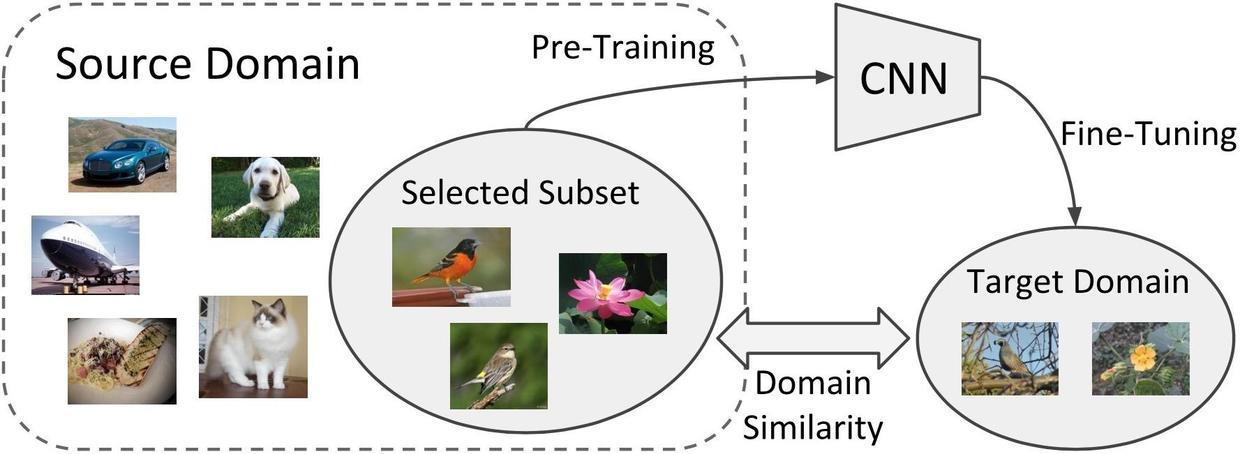## 框架

Jeremy 说这话，不是为了夸自己——因为他甚至做了个 MOOC 出来。他自己评价，说目前 fast.ai 的易用性依然不算成功。但在我看来， fast.ai 是目前把易用性和功能都做到了极致的深度学习框架。

## 数据

Jeremy 在 MOOC 中提到，如果你打算让机器通过数据来学习，你需要提供3样东西给它，分别是：

• 数据（Data）
• 模型结构（Architecture）
• 损失度量（Loss Metrics）

## 环境

fast.ai 官方，给出了以下5种云计算平台使用选项：

``````git clone https://github.com/wshuyi/demo-image-classification-fastai.git
``````

``````jupyter lab
``````

## 载入

``````from fastai import *
from fastai.vision import *
from fastai.core import *
``````

``````path = Path('imgs')
``````

``````data = ImageDataBunch.from_folder(path, test='test', ds_tfms=get_transforms(), size=224)
``````

• 我们进行了数据增强（augmentation），也就是对数据进行了翻转、拉伸、旋转，弄出了很多“新”训练数据。这样做的目的，是因为数据越多，越不容易出现过拟合（over-fitting），也就是模型死记硬背，蒙混考试，却没有抓住真正的规律。
• 我们把图片大小进行了统一，设置成了 224 x 224 ，这样做的原因，是我们需要使用迁移学习，要用到预训练模型。预训练模型是在这样大小的图片上面训练出来的，因此保持大小一致，效果更好。

``````data.show_batch(rows=3, figsize=(10,10))
``````

## 训练

``````learn = ConvLearner(data, models.resnet34, metrics=accuracy)
``````

``````learn.fit_one_cycle(1)
``````

5秒钟之后，训练结束。

``````preds,y = learn.get_preds()
interp = ClassificationInterpretation(data, preds, y, loss_class=nn.CrossEntropyLoss)
``````

``````interp.plot_top_losses(9, figsize=(10,10))
``````

``````interp.plot_confusion_matrix()
``````

## 评估

``````preds,y = learn.get_preds(is_test=True)
``````

``````data.test_dl.dl.dataset.ds.x
``````

``````preds
``````

``````np.argmax(preds, axis=1)
``````

## 微调

``````learn.unfreeze()
learn.fit_one_cycle(3, slice(1e-5,3e-4))
``````

``````data.test_dl.dl.dataset.ds.x
``````

``````preds,y = learn.get_preds(is_test=True)
``````

``````np.argmax(preds, axis=1)
``````